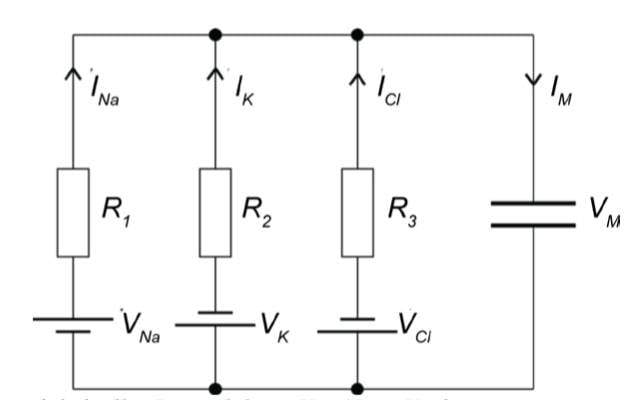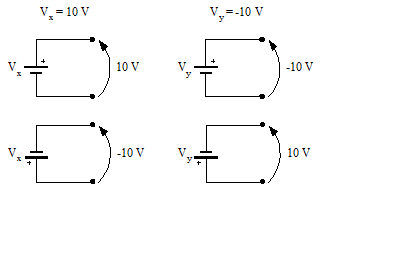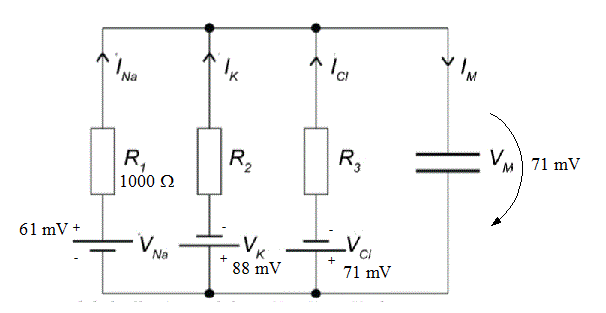# Capacitor in a circuitWe know that
- VM=VCl
- R1=1000.0 Ω
- IM=0
- VNa=61 mV
- VK=-88 mV
- VCl=-71 mV
Question is: what is R2?

I've written according to Kirchhoff's second law that
(1) VM + VNa - R1INa = 0
and (2) VM - VK - R2IK = 0.
We can also calculate that ICl=0.

With these equations and according to Kirchhoff's first law we can solve (using absolute values) that R2=128.789 Ω that is a correct answer. The problem is that I wonder why VM is always positive? Shouldn't it be negative in the first equation? If we go through capacitor and then Na-part of the circuit, the capacitor will have a negative charge at the bottom and a positive charge at the top because so has VNa. (Instead, if we continue to K or Cl part of the circuit, the capacitor will have a positive charge at the bottom and a negative at the top.) So the first equation would be -VM + VNa - R1INa = 0 but then we get an incorrect answer. I see that I must have misunderstood something but I don't know what is it.

Last edited:

## Answers and Replies

gneill
Mentor
Hi mini, Welcome to Physics Forums.

In future please be sure to retain and use the homework formatting template that is provided in the edit window when a new thread is created in the homework sections of the Forums. It's in the rules.

Can you clarify the signs associated with the various voltage values? The voltage source symbols shown in the diagram carry an implied polarity to be associated with whatever numerical value its parameter has. Changing the sign of the numerical value changes the orientation of the polarity that is presented to the circuit by the source. Consider the following picture:Note how the battery source presents whatever value the parameter has as the potential difference between its leads, with the longer bar having that value (with respect to the shorter bar). The "+" symbol, if present, is really superfluous for this schematic symbol since the orientation is implied by the shorter and longer bars that comprise it.

I note that in the circuit diagram the voltage sources have different orientations. This leads me to think that the orientation is intended to convey the direction of the polarities, and that the parameters really should be absolute values. It may seem a small thing, but when writing equations from a circuit diagram you really shouldn't have to know anything about the sign of any numerical values associated with the components. Otherwise it would add a level of complexity to the process that is error prone and difficult to sort out.

So, correct me if I'm wrong, but my interpretation of the scenario would assign voltage values to the circuit as depicted here:I note that in the original diagram no indication was given as to how to interpret the polarity orientation of ##V_M##. That's poor practice on the part of the problem author as a different solution will be found for either interpretation. Since it seems that they intend for ##I_{Cl}## to be zero, then the orientation would have to be as I've indicated above if ##V_M = V_{Cl}## as stated in the problem.

•mini and EM_Guy
andrevdh
Homework Helper
The fact that VM = VCl tells us that ICl = 0
since VM is the voltage over the parallel circuit.

I would assume that the top side of the capacitor is the positive side.

For equation 1, I get:
##V_m - V_{Na} + I_{Na}R_1 = 0## (KVL)

Equation 2:
##V_m + V_k + I_k R_2 = 0## (KVL)

Equation 3:
##V_m + V_{cl} + I_{Cl} R_3 = 0## (KVL)

Also, this is DC. I'm assuming that we are in steady state (since we are given that ##I_m = 0##). That is, the capacitor is essentially an open circuit.

Unknowns: ##I_k, I_{Cl}, R_2, R_3##

We need one more equation.

How about:

##V_k + R_2 I_k = V_{Cl} + R_3 I_{Cl}## (KVL)

4 equations, 4 unknowns. Plug and chug.

You need to pay very careful attention to your signs when you set up these equations.

Thank you for helping! I think gneill must be right: the signs of voltage values (that are actually potential values, don't know whether it matters here) must tell us only the direction of the polarities. The introduction of this task is really long and it isn't in English so I thought it'd be enough to tell the given values...
I think that this is also a very good point that I had forgotten to think about:
Since it seems that they intend for ICl to be zero, then the orientation would have to be as I've indicated above if VM = VCl as stated in the problem.

I think this is now clear for me, thank you!

(PS. I'm sorry, I was in a bit hurry so I thought I was using the template, I'll be more careful next time!)